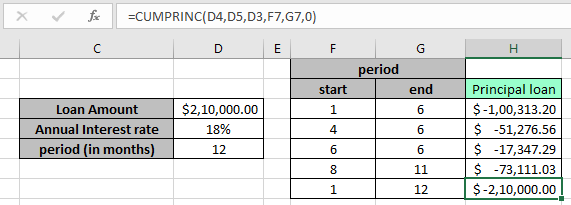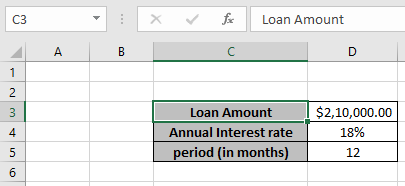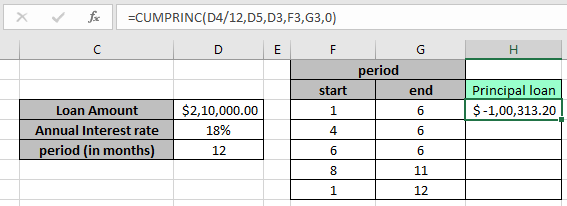# Calculating Total Annual Payment Based on Cumulative Monthly PaymentsIn this article, we will learn How to Calculate Total Annual Payment Based on Cumulative Monthly Payments in Excel.

Scenario:

While working with loan amounts and related queries, we usually don't understand how to calculate the monthly, quarterly, semi-annual or annual principal amount. The lender uses formula based on your chosen terms and conditions. Excel lets you access that formula using the CUMPRINC function. Understand more about how to calculate principal paid between the start and end period.

formula to return annual payment formula in Excel

CUMPRINC function in Excel returns the cumulative principal paid between two given periods. The function takes the rate, total period of loan and Loan amount as argument. Principal amount is The amount of money one borrows.

CUMPRINC Formula:

 =CUMPRINC (rate, nper, pv, start_period, end_period, type)

rate : The interest rate per period.

nper : The total number of payments.

pv : the loan amount

start_period : start month for the period

end_period : end month for the period

type : The timing of the payment, either at the beginning or end of the period. Numbers 0 or 1 represent the payment date. The number 0 represents payment at the end of the period, and the number 1 represents payment at the beginning of the period.

Example :

All of these might be confusing to understand. Let's understand how to use the function using an example. Here we have a loan amount from Mr X. Mr X borrowed a 1 year (12 payments) loan on the following terms.Mr X will pay the \$2,47,000, the total amount including principal amount and Interest amount via paying monthly payment approx \$21,000. Mr X wouldn't understand how much principal amount is paid over the first six months. Use the formula to calculate principal paid from 1st month to 6th month.

Use the formula :

 =CUMPRINC(D4/12,D5,D3,F3,G3,0)

Here

D4 : rate/12, annual rate divided by 12 for monthly payments.

D5 : total number of payments

D3 : total loan amount

F3 : start period

G3 : end period

0 : payment at the end of periodAs you can see, \$ 1,00,313 is the principal amount paid in the first six months. You must be wondering why there the resulting amount is with a negative sign, As we the loan amount or borrowed amount is positive so the amount returned comes with a negative sign.

Now change the start period and end period argument in the formula. To calculate the amount varying with different intervals of time.As you can see we get different amount values for different years.

Here are some observational notes using the formula/method explained above.

Notes:

1. Use as much arguments using cell reference which helps in error reduction
2. Type argument is not default in this function, throws an error "you've entered too few arguments for this function", If argument is not used.
3. The formula only works with numbers only, returns #VALUE! Error if any argument is non-numeric.
4. Do not use negative numbers in the argument as the formula generate #NUM! Error.
5. If you make monthly payments on a four-year loan at an annual interest rate of 12 percent, use rate/12 for monthly rate. Use rate directly 12%, if calculating for annual payments
6. If the start period < 1, end period < 1 or Start period > end period, the formula returns #NUM! Error.
7. Type argument other than 0 or 1, the formula returns #NUM! error.

Hope this article about How to Calculate Total Annual Payment Based on Cumulative Monthly Payments in Excel is explanatory. Find more articles on calculating values and related Excel formulas here. If you liked our blogs, share it with your friends on Facebook. And also you can follow us on Twitter and Facebook. We would love to hear from you, do let us know how we can improve, complement or innovate our work and make it better for you. Write to us at info@exceltip.com.

Related Articles :

Simple interest formula in Excel : Calculate the simple interest amount given the present or principal amount, rate in annum & period in years.

How to calculate interest on a loan : monthly instalment on a loan amount using the PMT function having principal amount or loan amount, interest rate per month and the period of payment.

How to use the RATE Function in Excel : RATE function is used to find the Interest rate of the data set in Excel. It requires the following arguments to calculate the interest rate.

How to use the PV function in Excel : PV function returns the present value of the fixed amount paid over a period of time at a constant interest rate.

How to use the NPV function in Excel : NPV function is used to find the net present value of the data set in Excel.

How to use the FV function in Excel : FV function in Excel returns the future value of the present amount having interest rate over a period.

Popular Articles :

How to use the VLOOKUP Function in Excel : This is one of the most used and popular functions of excel that is used to lookup value from different ranges and sheets.

How to use the IF Function in Excel : The IF statement in Excel checks the condition and returns a specific value if the condition is TRUE or returns another specific value if FALSE.

How to use the SUMIF Function in Excel : This is another dashboard essential function. This helps you sum up values on specific conditions.

How to use the COUNTIF Function in Excel : Count values with conditions using this amazing function. You don't need to filter your data to count specific values. Countif function is essential to prepare your dashboard.

Terms and Conditions of use

The applications/code on this site are distributed as is and without warranties or liability. In no event shall the owner of the copyrights, or the authors of the applications/code be liable for any loss of profit, any problems or any damage resulting from the use or evaluation of the applications/code.